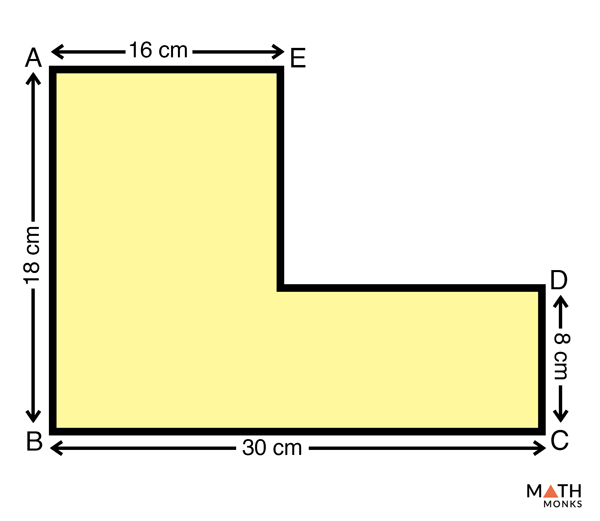# Area of Polygon

The area of a polygon is the total space enclosed within the shape. The measurement is done in square units.

As we know, a polygon can be regular or irregular. Regular polygons have a definite dimension to their sides, and thus their areas are easy to calculate compared to irregular polygons where the sides have no fixed dimension. Let us learn the basic approach for determining the area of both types, separately.

## How to Find the Area of a Regular Polygon

To determine the surface area of regular polygons with n sides (where each side is represented as ‘s’), we use the formula given below:

Find the area of a regular polygon with perimeter of 44 cm and apothem length of 10 cm.

Solution:

As we know,
Area (A) = ½ x p x a, here p = 44 cm and a = 10 cm
= ½ x 44 x 10 cm2
= 220 cm2

Finding the area of regular polygon when the SIDE and APOTHEM are known.

Calculate the area of a regular pentagon with side 12 cm and apothem of 7.5 cm.

Solution:

As the polygon is a pentagon having five sides, where each side (s) measures 12 cm, its perimeter (p) is = (5 x s) = (5 x 12) = 60 cm
Now, as we know,
Area (A) = ½ x p x a, here p = 60 cm and a = 7.5 cm
= ½ x 60 x 7.5 cm2
= 225 cm2

## How to Find the Area of an Irregular Polygon

For irregular polygons, we divide the polygon into two or more regular polygons, find the individual area of each such polygon, and then add them to get the area of the total figure.

Let us take an example to understand the concept better.Find the area of the irregular polygon ABCDE with given side measurements. (Trick: Divide the polygon into two rectangles)Solution:

To solve the given problem, let us divide the given figure into two rectangles ABFE and GFDC.
Now, as we know,
Area of a rectangle = l x b, here l = length and b = breadth
In rectangle ABFE, lengths (AB = FE) = 18 cm and breadths (AE = BF) = 16 cm
Thus, area of rectangle ABFE = (18 x 16) = 288 cm2
Similarly, in rectangle GFDC, lengths (GF = DC) = 14 cm and breadths (GD = FC) = 8 cm
Thus, area of rectangle GDFC = (14 x 8) = 112 cm2
Since, area of polygon ABCDE = area of rectangle ABFE + area of rectangle GDFC
= (288 + 112) cm2
= 400 cm2

## Finding the Area of Some Common and Basic Polygons

If we are looking to find the area of other basic polygons such as triangle, rectangle, parallelogram, rhombus, trapezoid, kite, pentagon, and hexagon, we can also use their standard formulas that are given below: